### MARTIAN METEORITES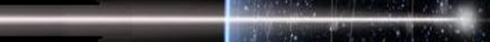INTRODUCTION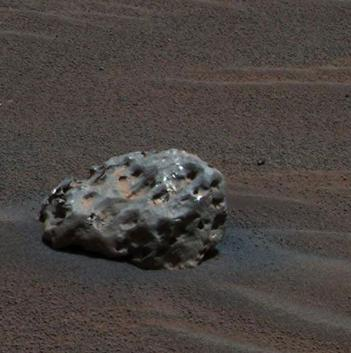The image at left is a picture of the Martian surface taken by the NASA rover Opportunity. The item of interest in the image is an iron meteorite, called Heat Shield Rock, with a length in the longest direction of 31 cm. This gives it a mass of several tens of kilograms. At the time of writing (2012) NASA rovers had found 6 meteorites. A simple calculation of the search area of the rovers as a fraction of the Martian surface gives an estimate of around one billion meteorites just waiting to be picked up from the Martian surface! The big problem however is that the meteorite in the image should not be there!! We will explain why in the following notes.

TERMINOLOGY

The following definitions are from IAU Commission 22.

 METEOROID - the body in space ( less than about 10 metres in size ) METEOR - the phenomenon of a meteoroid entry through the atmosphere ( producing light, heat and ionisation ) - it never should be used to refer to the body producing the meteor METEORITE - if any part of the meteoroid survives the atmospheric entry process and deposits something on the surface - a body on a planet

CLASSIFICATION OF METEORIC PHENOMENA

The sizes in the following table are for the Earth.

METEOROID SIZEPHENOMENA / REGIME

Very small
(sub mm)

Declerated without ablation
---> Micrometeorite ( no meteor)

Small
(mm - cm)

Meteoroid totally ablated
---> Meteor (ablation products)

Medium
(10 kg - 10 ton)

Ablation not total
---> Meteor/Fireball - Meteorite

Large
(> 100 ton)

Insignificant ablation, hypervelocity
impact - total destruction
---> Fireball - Crater (no meteorite)

METEORIC PHENOMENA ON DIFFERENT PLANETS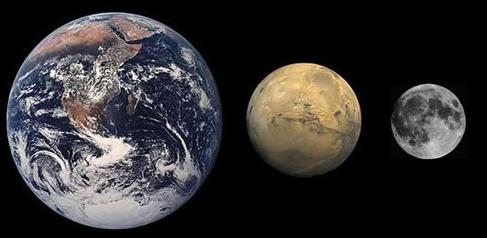The following table shows the occurrence of meteors, meteorites and craters on the Earth, Mars and the Moon.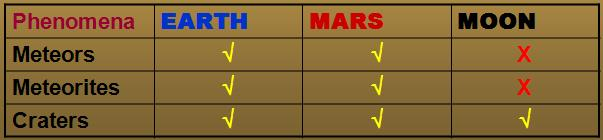The absence of an atmosphere denies production of the meteor phenomenon and allows all meteoroids to hit the surface at hypervelocity, which will create a crater and vapourise the incident projectile.

An atmosphere on the other hand, even one of low density, will allow the occurrence of meteors and meteorites.

THE METEOR REGIME

The two graphs below show the visual light curves that result from meteoroids with a mass of one gram but with varying velocities (from 20 to 65 km/sec) as they enter the atmospheres of Earth and of Mars. The plots show the variation in apparent magnitude (as seen by an observer directly beneath the meteor) with height.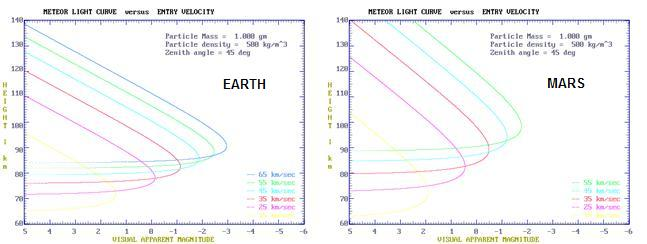The curves are remarkably similar. Maximum brightness occurs between about 70 and 100 km. What is interesting is that the meteor becomes visible at a higher altitude in the Martian atmosphere, even though the density of the Martian atmosphere at the surface of the planet is about 80 times less than is the surface atmospheric density on Earth. Still, what this shows us is that the meteor phenomenon for small bodies is remarkably similar between the two planets.

LARGER METEOROIDS

For a one gram particle there is no resulting meteorite, either on the Earth or on Mars. There is total ablation of the meteoroid into dust particles that will slowly drift down through the atmosphere.

The result is however, quite different for larger bodies that might produce a meteorite. On the Earth, a initial iron meteoroid of 100 kg might be expected to 'drop' a meteorite of about 2% its mass, that is, a two kilogram body on the surface. The parameters of the flight of such a body are shown in the graph below.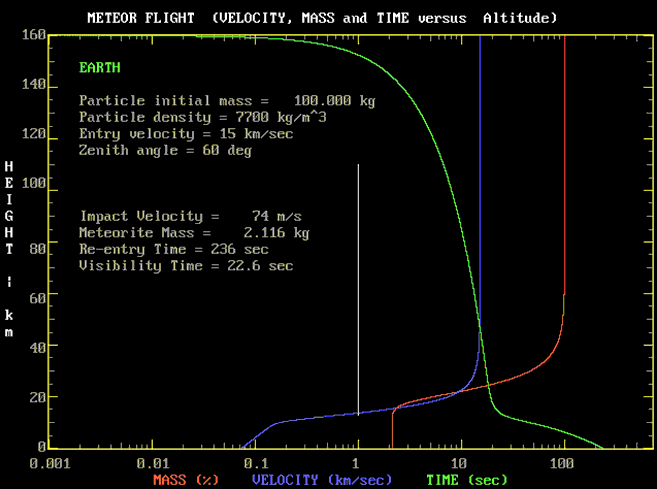We find that the circumstances or parameters required to produce a Martian meteorite are quite different.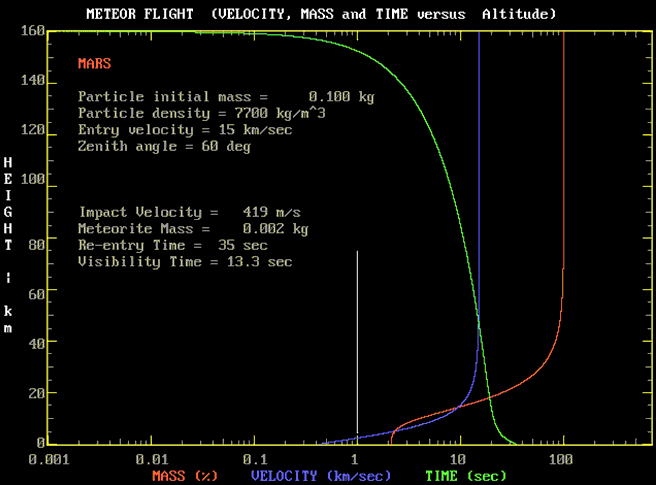We only have to increase the mass of the meteoroid by ten (to 1 kg) and we have a hypervelocity impact which both vapourises the meteoroid and creates an impact crater on the Martian surface.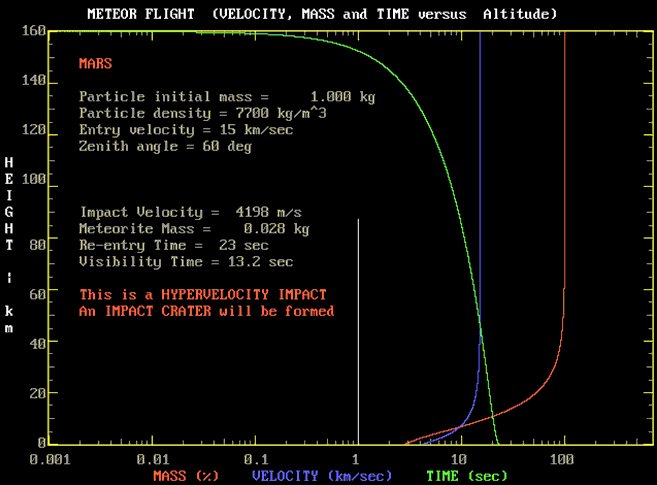ATMOSPHERIC BRAKING

The difference between a hypervelocity impact and a lower velocity impact in which a meteorite survives is all due to the amount of atmosphere that the meteoroid encounters.

The principle of atmospheric braking states that the mass of atmosphere encountered in an entry or reentry process will brake an equal mass of meteoroid. A useful formula which quantitatively expresses this principle allows us to determine the maximum mass that will not vapourise the meteoroid:

mcrit = 9 π ρo2 H3 / ( 16 ρm2 )
where
mcrit is the critical mass of the meteoroid
π ~ 3.14
ρo is the surface air density of the planet
H is the scale height of the atmosphere
ρm is the density of the meteoroid
The table below gives the surface atmospheric density and scale height for Earth and Mars (the scale height is the height at which the air density has fallen to a fraction 1/e = 0.37 its value at the surface).

Parameter EARTH MARS
Surface Atmospheric Density1.25 kg m-30.0156 kg m-3
Atmospheric Scale Height7000 m10,000 m

The next table gives values of critical mass for meteoroids entering both the terrestrial and martian atmospheres. A cometary meteoroid might have a density of 500 kg m-3, a stony meteoroid a density of 3500 kg m-3 and an iron meteoroid a density of 7700 kg m-3.

Meteoroid
Density
(kg m-3)
EARTH
Critical Mass
(ton)
MARS
Critical Mass
(kg)
500450027
100011007
20002801.7
4000700.4
8000180.1

The above figures are for vertical incidence. For near horizontal entry, they will be increased by ~ 10.

IRON METEORITE SIZES

Using a formula to compute the diameter (d) of a spherical body of density ρ from its mass (M):

d = 3√[ 6 M / π ρ ]
and taking the density of an iron meteorite as 7700 kg m-3 we can calculate the diameter of iron meteoroids of various masses:

MASS
(kg)
DIAMETER (mm)
0.129
0.237
0.550
163
279
5107
10135
20171
50232
100292

An iron meteoroid with a critical mass (for vertical incidence) of 0.1 kg will only be 3 cm in size. Even a near horizontal entry would not leave a 1 kg meteorite (~6.5 cm) on the surface, let alone a larger one. It is simply not possible for a single meteor process on Mars with its current atmospheric density to result in an iron meteorite with the mass of the Heat Shield Rock.

An alternative explanation is that during a hypervelocity collision not all of the large impactor is always vapourised, and some of the material is thrown out of the impact crater to land at a much lower velocity elsewhere on the surface. However, if this is the case, we would not expect to find the typical regmaglypts ('thumbprints') all over the surface of such an ejecta (as we seem to see on Heat Shield Rock).

CONCLUSION

The presence of heavy iron meteorites on the Martian surface probably implies a much denser Martian atmosphere in the past.

And from the lack of really significant weathering of the iron meteorites discovered, maybe not all that long ago (in areological terms)??

POSTSCRIPT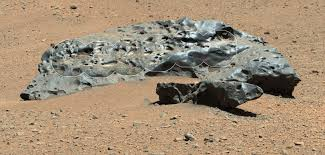Since the above was written, the NASA Mars rover named Curiosity has discovered (2016) a very large iron meteorite (named Lebanon A & B - there are two components) that is over two metres in length!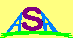Australian Space Academy